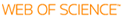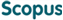BROWSE

Related ResearcherJung, Chang-Yeol
Analysis and computational methods Lab
Research Interests
• Analysis, singular perturbations, uncertainty, numerical methods

FINITE VOLUME APPROXIMATION OF TWO-DIMENSIONAL STIFF PROBLEMS

Cited 0 times inCited 4 times inTitle
FINITE VOLUME APPROXIMATION OF TWO-DIMENSIONAL STIFF PROBLEMS
Author
Keywords
Asymptotic analysis; Boundary layers; Correctors; Finite volume methods; Singularly perturbed problems; Stiff problems
Issue Date
2010-09
Publisher
ISCI-INST SCIENTIFIC COMPUTING & INFORMATION
Citation
INTERNATIONAL JOURNAL OF NUMERICAL ANALYSIS AND MODELING, v.7, no.3, pp.462 - 476
Abstract
Continuing an earlier work in space dimension one, the aim of this article is to present, in space dimension two, a novel method to approximate stiff problems using a combination of ( relatively easy) analytical methods and finite volume discretization. The stiffness is caused by a small parameter in the equation which introduces ordinary and corner boundary layers along the boundaries of a two-dimensional rectangle domain. Incorporating in the finite volume space the boundary layer correctors, which are explicitly found by analysis, the boundary layer singularities are absorbed and thus uniform meshes can be preferably used. Using the central difference scheme at the volume interfaces, the proposed scheme finally appears to be an efficient second-order accurate one.
URI
ISSN
1705-5105
Appears in Collections:
SNS_Journal Papers
Files in This Item: